Technical Article

# Determining Series RLC Circuit Resonance Frequency

May 02, 2023 by Amna Ahmad

## Learn how to determine the resonance frequency, the component voltages, and current levels at various frequencies in a series RLC circuit.

A series RLC circuit contains a resistor (R), an inductor (L), and a capacitor (C) connected in series. Resonance in a series RLC circuit occurs when the reactive effects of the inductor and capacitor cancel each other out, resulting in a purely resistive circuit. At resonance, the circuit exhibits some interesting properties, such as a maximum current and a minimum impedance. Understanding the phenomenon of resonance in a series RLC circuit is essential in designing and analyzing many electronic devices and systems.

### Frequency Effect on Circuit Impedance

The series-connected RLC circuit shown in Figure 1(a) has an impedance of

$Z=R+j{\big(}X_{L}-X_{C}{\big)}\,(1)$

Or

$Z=\sqrt{R^{2}+X^{2}}\angle tan^{-1}{\Big(}\frac{X}{R}{\Big)}$

Because XL=2πfL and XC=1/(2πfC), the actual impedance of the circuit depends on the frequency of the alternating supply, as illustrated in Figure 1(b). Figure 2 shows the values of jXL and -jXC plotted versus supply frequency. It is seen that because XL is directly proportional to frequency, the inductive reactance increases linearly from zero with the increase in frequency. However, the capacitive reactance is inversely proportional to the frequency, and at low frequencies, XC is infinitely large. As the frequency increases, XC rapidly decreases from infinity but never becomes zero. The resulting graph of (-jXC) versus frequency is curved, as illustrated.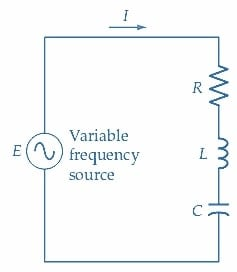##### (a) Series RLC circuit with a variable frequency source.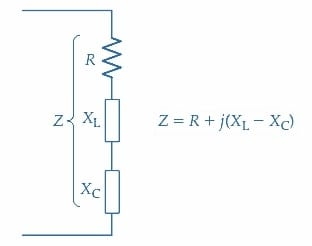##### Figure 1. In a series RLC circuit with a variable frequency source, XL becomes equal to XC at a particular frequency, known as the resonance frequency. The circuit impedance is then equal to R. Image used courtesy of Amna Ahmad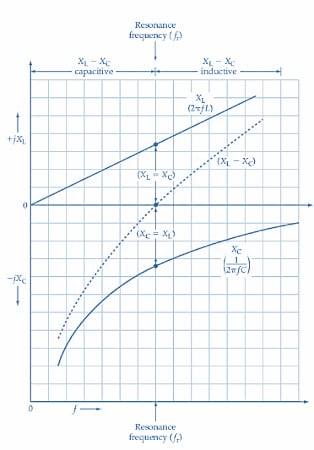##### Figure 2. A plot of +jXL and -jXC versus frequency for a series RLC circuit shows that at a particular frequency (fr), XL = XC, and consequently (XL = XC) = 0. The frequency fr is known as the resonance frequency for the circuit. Image used courtesy of Amna Ahmad

The total reactance of the series-connected RLC circuit is (XL - XC), and the graph of this quantity is shown by the dashed line in Figure 2. At low frequencies, it is clear that XL is much smaller than XC, and thus the total reactance is largely capacitive. At the higher frequencies, where XL is much larger than XC, the total reactance becomes largely inductive. At one frequency, identified as fr on the graph, XL and XC are numerically equal. Consequently, the impedance of the series RLC circuit at fr becomes

$Z=R+j{\Big(}X_{L}-X_{C}{\Big)}=R+j(0)$

Or

$Z=R$

When this occurs, the circuit is said to be in a state of electrical resonance, and the frequency at which XL equals XC is known as the resonance frequency (fr).

The resonance frequency for a series RLC circuit is defined as the frequency at which XL=XC.

Referring again to Figure 2, it is seen that at frequencies above and below resonance, the reactance (X = XL - XC) has a large value, which is either inductive or capacitive. So, when the circuit impedance (Equation 1) is plotted versus frequency, it is found that the impedance has a high value above and below the resonance frequency, and at resonance, it dips to its minimum value of Z = R [see Figure 3(a)].

A series RLC circuit has minimum impedance at the resonance frequency.

### Current at Resonance

The current in the series RLC circuit is determined from

$I=\frac{E}{R+j{\Big(}X_{L}-X_{C}{\Big)}}\,(2)$

Or

$|I|=\frac{E}{\sqrt{R^{2}+{\Big(}X_{L}-X_{C}{\Big)}^{2}}}$

Where I is the numerical value of the current without reference to its phase angle. Thus, at resonance, when XL=XC, the current equation becomes

$I=\frac{E}{R}$

A typical graph of current versus frequency for the series RLC circuit is shown in Figure 3(b). The current is a minimum at frequencies above and below resonance but peaks sharply at resonance as the impedance falls to a minimum. The current/frequency graph is sometimes referred to as the frequency response curve of the circuit.

### Phase Angle

As noted from Figure 2, the impedance of the series RLC circuit is largely capacitive at frequencies well below resonance. This means the circuit current leads the applied voltage by a phase angle of approximately 90°. Conversely, because the impedance is largely inductive at frequencies much greater than the resonance frequency, the phase angle of the current above resonance is approximately -90°. The graph of phase angle versus frequency for the series RLC circuit, as in Figure 3(c), shows the 90° leading phase angle at low frequencies, changing to 0° at the resonance frequency, and moving to a 90°lagging phase angle above fr.##### (a) Graph of impedance versus frequency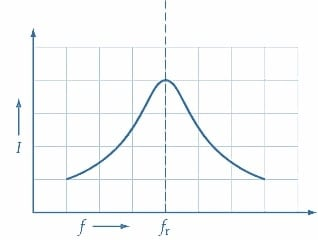##### (b) Graph of current versus frequency### Resonance Frequency

The frequency at which resonance occurs is easily calculated by equating XL and XC:

$X_{L}=2\pi f_{r}L$

$X_{C}=\frac{1}{2\pi f_{r}C}$

So

$2\pi f_{r}L=\frac{1}{2\pi f_{r}C}$

Giving

$f_{r}=\frac{1}{2\pi\sqrt{LC}}\,(3)$

When L and C are in henrys and farads, respectively, Equation 2 gives fr in hertz.

Example 1

Determine the resonance frequency for the series RLC circuit in Figure 4. Also, calculate the circuit currents at 0.25fr, 0.5fr, 0.8fr, fr, 1.25fr, 2fr, and 4fr. Plot a graph of current versus frequency to a logarithmic base.

Solution

Equation 3

$f_{r}=\frac{1}{2\pi\sqrt{LC}}=\frac{1}{2\pi\sqrt{85\mu H\times298pF}}=1MHz$

Equation 2

$|I|=\frac{E}{\sqrt{R^{2}+(X_{L}-X_{C})^{2}}}$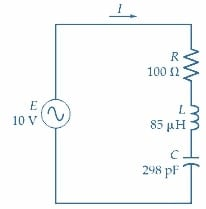##### Figure 4. Circuit for Example 1. Image used courtesy of Amna Ahmad

The values of the various quantities are tabulated below for each frequency, and the graph of current versus frequency is plotted in Figure 5.

 f(Hz) XL(Ω) XC(Ω) (XL-XC)Ω Z I (mA) 250k 134 2136 -j2k ≈2k 5 500k 267 1068 -j801 ≈807 12.4 800k 427 668 -j241 ≈261 38.3 1M 534 534 0 100 100 1.25M 668 427 +j241 ≈261 38.3 2M 1068 267 +j801 ≈807 12.4 4M 2136 134 +j2k ≈2k 5

The graph of current versus frequency in Figure 5 clearly shows that the current rises to a maximum at the resonance frequency and falls off sharply at frequencies above and below resonance. Note from the table that when the frequency is doubled from 250 kHz to 500 kHz, the current increases from 5 mA to 12.4 mA. Also note that above resonance, the current decreases from 12.4 mA to 5 mA when the frequency is doubled from 2 MHz to 4 MHz. The same sort of corresponding current ratios is evident for other frequency changes above and below the resonance frequency. It is seen that the ratio of current to frequency is logarithmic, and so a logarithmic frequency scale is used on the graph.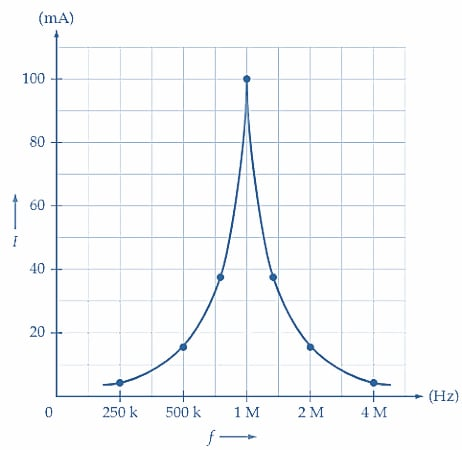### Resonance Rise in Voltage

The voltage across the resistance in a series RLC circuit is VR=IR, and at resonance VR equals the supply voltage [see Figure 6(a)]. As illustrated, the capacitor and inductor voltages are:

$V_{C}=IX_{C}\,(4)$

And

$V_{L}=IX_{L}\,(5)$

When the capacitor voltage levels and inductor voltage levels are calculated for various frequencies and plotted to a logarithmic frequency scale, it is found that the VC and VL frequency response curves are similar in shape to the circuit current-versus-frequency graph. It is also found that

VL and VC at resonance can be several times greater than the supply voltage.

This effect is called the resonance rise in voltage.

Figure 6(b) shows that the waveforms of VL and VC are in phase opposition at resonance. Thus, the instantaneous levels of VL and VC cancel each other, and all the supply voltage appears across R.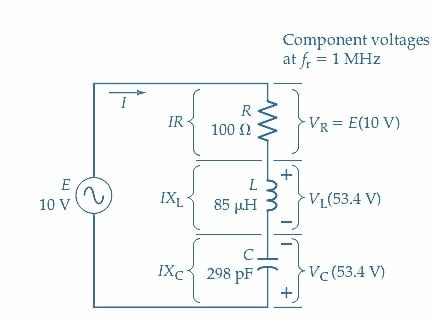##### (a) Circuit and voltages at resonance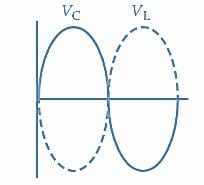##### Figure 6. In a series RLC circuit, the resonance frequency VR=E, and VL and VC are larger than E. VL, and VC are in phase opposition. Image used courtesy of Amna Ahmad

Example 2

For the series RLC circuit in Figure 6 (as in Example 1), determine the capacitor and inductor voltages at each frequency. Also, plot the graphs of VR, VC, and VL versus frequency.

Solution

$V_{C}=IX_{C}\,and\,V_{L}=IX_{L}\,and\,V_{R}=IR$

The quantities are tabulated for each frequency, and the graphs are plotted in Figure 7. Note from the graph that VC>VL below resonance, and VL>VC above resonance.

 F(Hz) VR(V) VL(V) VC(V) 250k 0.5 0.67 10.7 500k 1.24 3.3 13.2 800k 3.83 16.4 25.5 1M 10 53.4 53.4 1.25M 3.83 25.5 16.4 2M 1.24 13.2 3.3 4M 0.5 10.7 0.67

Example 2 demonstrates that at resonance, the voltages across the capacitor and inductor become much greater than the supply voltage. The voltage across the resistance (VR) is, of course, in phase with the circuit current, while VL leads the current by 90° and VC lags the current by 90°. This is illustrated in the phasor diagram in Figure 8(a). From the phasor diagram, it is clear that VL and VC cancel at resonance and the supply voltage is applied across the resistance.##### Figure 7. Graphs of capacitor voltage VC, inductor voltage VL, and resistor voltage VR versus frequency (to a logarithmic base) for a series RLC circuit. At the resonance frequency, VC and VL become much greater than the supply voltage. Image used courtesy of Amna Ahmad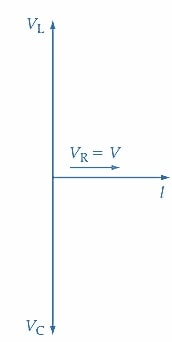##### (a) Phasor diagram of a series RLC circuit at resonance.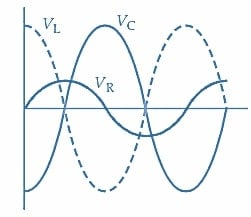### Energy Transfer Between L and C

The waveforms of VL, VR, and VC at the resonance frequency are shown in Figure 8(b). It is evident that as VL grows positively, VC becomes more negative, and vice versa. The positive peak of VL occurs at the same instant as the negative peak of VC, and the positive peak of VC corresponds in time with the negative peak of VL. Also, note that the positive and negative voltage peaks across the resistance coincide with the instant when VL and VC are zero. The information derived from these waveforms is that energy is stored in the resonant circuit. The energy is continuously transferred from the inductor to the capacitor and back again at the resonance frequency. The resonance frequency is the only frequency at which this energy transfer can take place for the particular values of the capacitor and inductor involved. Note, however, that the energy storage and energy transfer between reactive components occurs only when there is an energy input at the resonance frequency.

### Takeaways of Determining Resonance Frequency

When a series-connected RLC circuit has the frequency of its alternating supply voltage varied, XL = XC at a particular frequency. The two reactances cancel, and the result is the series impedance of the circuit has a minimum value of Z=R. The circuit is in a state of resonance. The frequency at which this occurs is the resonance frequency. Because the impedance is a minimum, the series current is a maximum, and there is a rise in the voltage developed across L and C.

To catch up on the other articles in this series, follow these links:

Featured image used courtesy of Adobe Stock